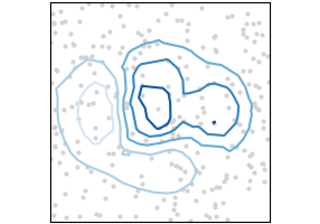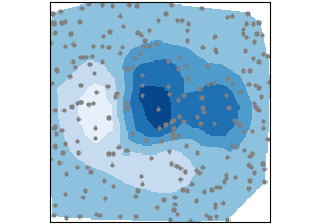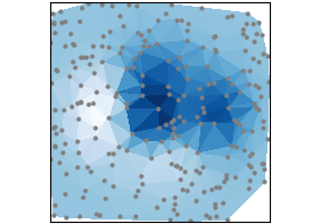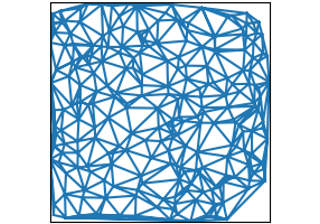# Irregularly gridded data#

Plots of data $$Z_{x, y}$$ on unstructured grids , unstructured coordinate grids $$(x, y)$$, and 2D functions $$f(x, y) = z$$.tricontour(x, y, z)

tricontour(x, y, z)tricontourf(x, y, z)

tricontourf(x, y, z)tripcolor(x, y, z)

tripcolor(x, y, z)triplot(x, y)

triplot(x, y)